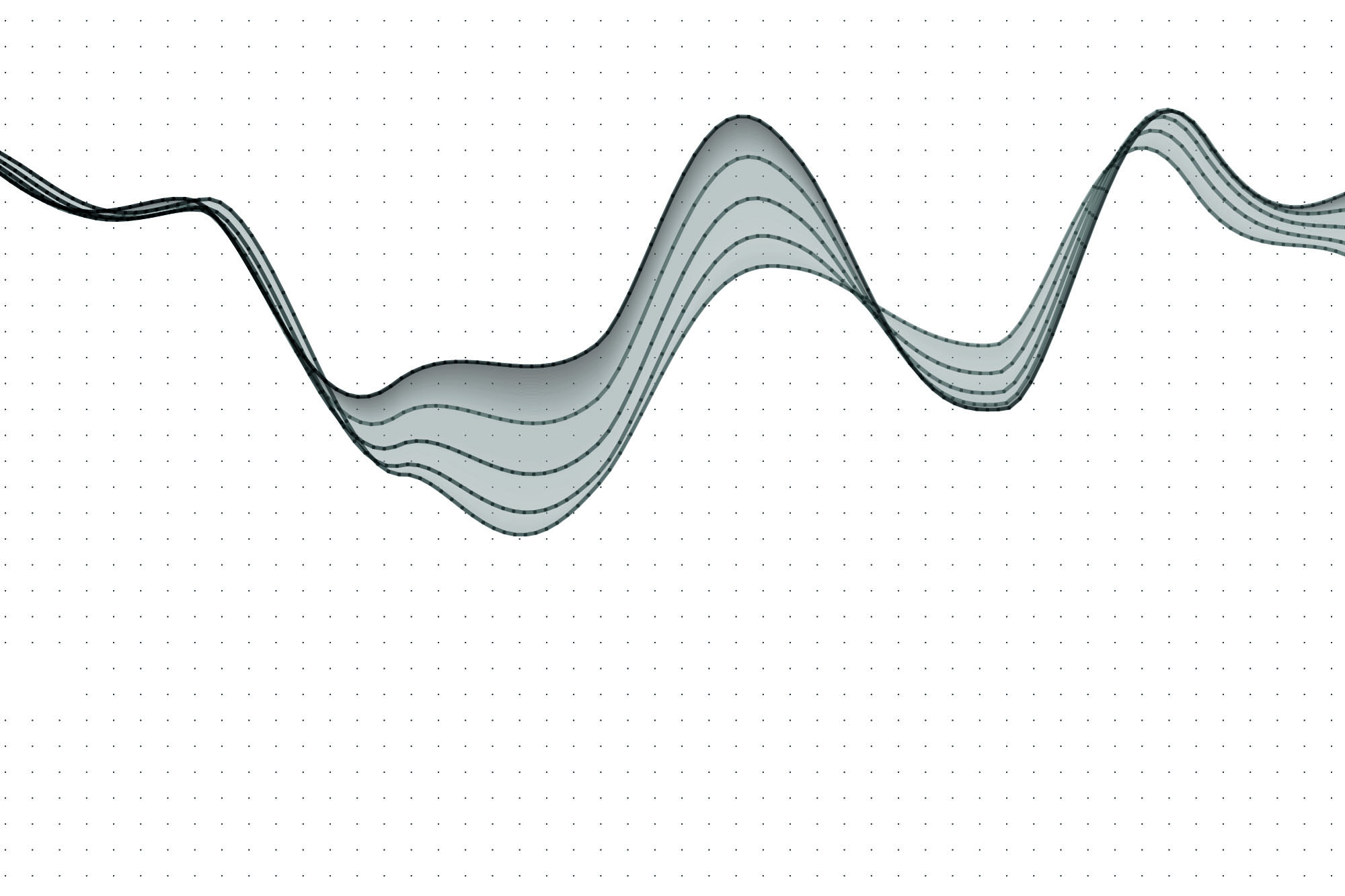# Demystifying the World of Machine Learning

Neural Networks are a popular data science tool being applied to engineering problems. They work by linking a given set of input variables to a given set of output variables. What happens in the middle might appear as a black box system, it certainly did to me at first, as they are not based on the physical laws that drive most other engineering analysis tools. In this article, we’re going to shine some light into that black box. I’ll share an overview of how neural networks work, how to assess their error and uncertainty and the levers you can pull within that black box, in order to improve the process and enable robust engineering.

Within a neural network, the inputs and outputs are connected by a spider web of linkages, all of varying weights. You can alter the structure and size of the web; how many layers there are between the inputs and outputs, and how many points there are per layer – the neurons. It’s not a physical model of the data, it’s a purely mathematical representation of the data. The neural network learns on a given data set you provide it with. It then repeatedly adjusts these weights until the output variables can be reproduced for any combination of inputs. For example, you can connect the power output of a wind turbine to the input operating conditions and geometry of the turbine blades. It’s an incredibly quick and effective way of generating models of complex relationships.

How accurate is this process? Most measurement techniques have some error and neural networks are no different. You can assess the accuracy of a neural network by providing it with a new, previously unseen dataset and comparing the predicted output of the neural network to the known output from this new dataset. This gives a measure of the accuracy of the network; how close the output value is to the reference value e.g. The neural network predicts a power coefficient of the wind turbine for a given set of inputs is 0.305, and the measured value is 0.291. The accuracy is 0.014. It is common practise to validate new measurement techniques before using them, and this is also recommended for machine learning models.

The next important measure to assess for the model is its uncertainty. Precision is a measure of how spread the output values are around the average when you use a neural network to make repeat predictions. One method of calculating uncertainty for neural networks is to remove a percentage of the linkages in the web and recalculate the outputs. This is referred to as dropout. During training, every time the neural network adjusts its weights some of the linkages are intentionally removed and the final output is consequently slightly different each time. After repeating this loop 100 times, the neural network has a spread of answers for each output and once finished training the mean output is returned alongside the uncertainty range. For my wind turbine example, the uncertainty range on the power coefficient for a given variable is 0.305, lower limit 0.287 to upper limit 0.322. We define this uncertainty range as plus or minus double the standard deviation σ in the spread of values. This method of calculating the uncertainty range on a neural network provides a 4σ range and consequently you can be confident that the neural network prediction will lie within this range 95% of the time

For a process or measurement technique to be robust it needs to have a low variability. Having no variability at all is unrealistic, as such, most processes have limits within which some variability of the output is acceptable e.g. a power coefficient limited to between 0.25 and 0.45. A good neural network needs to have the mean output centred within the acceptable limits and the spread of the output needs to be well within these limits also. If both of these criteria are met, then great, you can be confident that your neural network is robust, and you can go on to use it for predicting the performance of new designs.

If these conditions are not met, then it requires a bit of tuning, like you would a power meter before first use. You can tune your network by adjusting some of those levers we met earlier - changing its shape, structure, dropout or the number of training loops. When tuning your neural network, the target is to have an uncertainty and error which matches that expected from the underlying dataset it is trained on, and this still requires a bit of engineering judgement.

Machine learning models are a useful way of representing complex nonlinear physics problems, but they need calibrating and tuning like any measurement device or computer aided engineering tool. Once this is done, you can be a little less ‘uncertain’ about using tools like neural networks, for making robust predictions.

No items found.